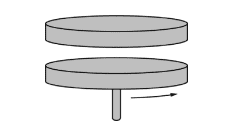# The inelastic collision between a disk and a rotating platform

Leo Liu
Homework Statement:
A FR question in 2019 AP Physics C Exam (Version 2)
Relevant Equations:
Rotational Kinetic Energy, Conservation of Angular Momentum
A disk is dropped on a platform rotating at a constant angular speed ##\omega_i## as shown below.The question asks whether the final kinetic energy of the platform is conserved. I understand the angular momentum is always conserved provided that the net torque is 0, so I wrote the following equation:
$$I_{platform} \omega_i = (I_{platform}+I_{disk}) \omega_f$$
From this I inferred that ##\frac 1 2 I_{platform} {\omega_i}^2 \neq \frac 1 2 (I_{platform}+I_{disk}) {\omega_f}^2##.

My questions are as follows: Why is the energy not conserved in the collision, and how is the energy dissipated?

#### Attachments

Last edited:

What force must act between the platform and the disk in order to equalise their angular velocities? Does this force dissipate mechanical energy into anything else?

Leo Liu
What force must act between the platform and the disk in order to equalise their angular velocities? Does this force dissipate mechanical energy into anything else?
I think it's kinetic friction because the angular speed of the platform decreases as the rotation of the disk speeds up. Am I right?

I think it's kinetic friction because the angular speed of the platform decreases as the rotation of the disk speeds up. Am I right?

Yes that's right.

And friction dissipates mechanical energy into thermal energy. Actually, the total work done by friction at the interface, ##W##, is the change in mechanical energy of the system. The change in thermal energy of the system is ##-W##. Energy is conserved... just not KE!

•Leo Liu